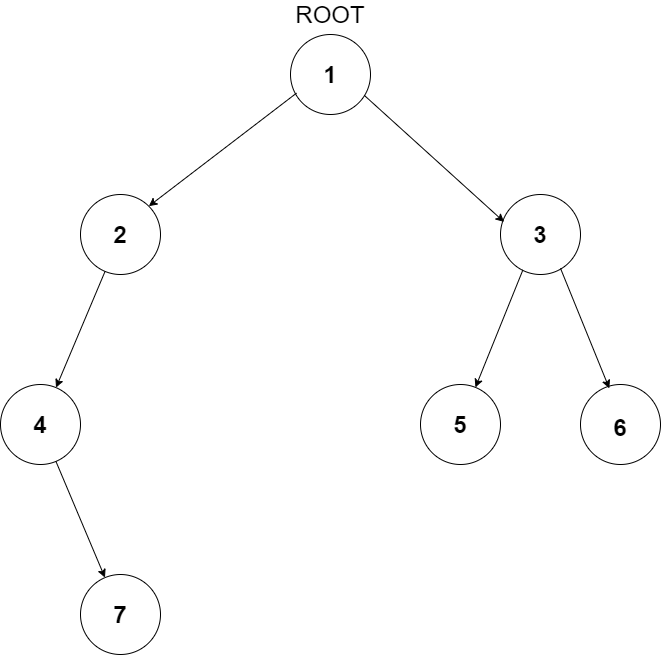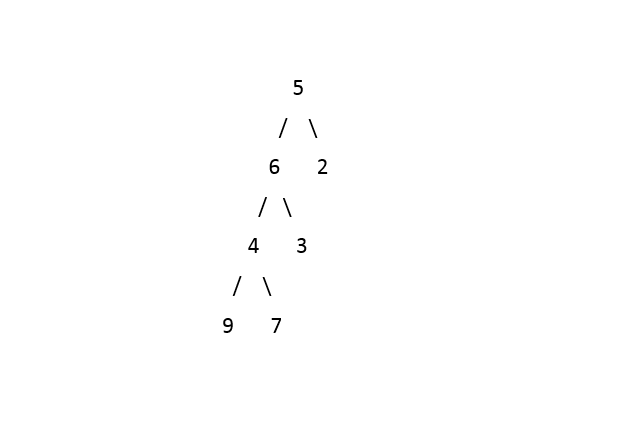Problem title
Difficulty
Avg time to solve

Count Frequency
Easy
15 mins
Left Sum
Easy
10 mins
Special Binary Tree.
Easy
15 mins
Integer To Roman Numeral
Easy
10 mins
First Unique Character in a String
Easy
15 mins
Find Pair Sum in Rotated and Sorted array
Easy
10 mins
Count Nodes within K-distance
Hard
10 mins
Sum of Two Elements Equals the Third.
Easy
10 mins
Detect Cycle In A Directed Graph
Moderate
30 mins
Number of balanced binary trees
Easy
10 mins11

# Distance between two nodes of a Tree

Difficulty: MEDIUM
Avg. time to solve
25 min
Success Rate
60%

Problem Statement

#### Distance between two nodes is defined as the minimum number of edges in the path from one node to another.

##### Input Format:
``````The first line of input contains an integer ‘T’ representing the number of test cases. Then the test cases follow.

The first line of each test case contains elements in the level order form, and all the node values in the given tree are unique. The line consists of values of nodes separated by a single space. In case a node is null, we take -1 in its place.

The second line of each test case contains two single-spaced integers, Node 1 and Node 2, representing the values of the two nodes.

For example, the input for the tree depicted in the below image would be:
````````````1
2 3
4 -1 5 6
-1 7 -1 -1 -1 -1
-1 -1
7 5
``````

#### Explanation:

``````First 5 lines represent the tree, and the last line contains the value of the nodes between which we have to find the distance.

Level 1:
The root node of the tree is 1

Level 2:
Left child of 1 = 2
Right child of 1 = 3

Level 3:
Left child of 2 = 4
Right child of 2 = null (-1)
Left child of 3 = 5
Right child of 3 = 6

Level 4:
Left child of 4 = null (-1)
Right child of 4 = 7
Left child of 5 = null (-1)
Right child of 5 = null (-1)
Left child of 6 = null (-1)
Right child of 6 = null (-1)

Level 5:
Left child of 7 = null (-1)
Right child of 7 = null (-1)

The first not-null node(of the previous level) is treated as the parent of the first two nodes of the current level. The second not-null node (of the previous level) is treated as the parent node for the next two nodes of the current level and so on.
The input ends when all nodes at the last level are null(-1). The last line contains the value of the two nodes (7 and 5) between which we have to find the distance.
``````
##### Note:
``````The above format was just to provide clarity on how the input is formed for a given tree.
The sequence will be put together in a single line separated by a single space. Hence, for the above-depicted tree, the input will be given as:

1 2 3 4 -1 5 6 -1 7 -1 -1 -1 -1 -1 -1
7 5
``````
##### Output Format:
``````For each test case, a single integer denoting the distance between the two nodes is printed. In case, if any of the nodes is not found in the tree, return -1.

The output for each test case is in a separate line.
``````
##### Note:
``````You do not need to print anything, it has already been taken care of. Just implement the give function.
``````
##### Constraints:
``````1 <= T <= 10 ^ 2
1 <= N <= 3 * 10 ^ 3
0 <= DATA <= 10 ^ 6 and DATA != -1

Where ‘T’ is the number of test cases, ‘N’ is the total number of nodes in the binary tree, and ‘DATA’ is the value of the binary tree node.

Time Limit: 1 sec.
``````
##### Sample Input 1:
``````2
5 6 2 4 3 -1 -1 9 7 -1 -1 -1 -1 -1 -1
7 2
1 3 -1 2 -1 -1 -1
2 4
``````
##### Sample Output 1:
``````4
-1
``````
##### Explanation of Sample Input 1:``````For the first test case, the distance between node 7 and node 2 will be 4 as there are 4 edges between these two given nodes, i.e. 7 -> 4, 4 -> 6, 6 -> 5, and 5 -> 2.

For the second test case, the answer will be -1 as node 4 is not present in the binary tree.
``````
##### Sample Input 2:
``````2
1 2 3 4 5 6 7 8 9 -1 -1 -1 -1 -1 -1 -1 -1 -1 -1
7 1
1 2 3 4 5 -1 -1 -1 -1 -1 -1
3 5
``````
##### Sample Output 2:
``````2
3
``````Console